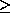Next: Null Values Up: SQL Previous: Set Operations

Aggregate Functions

1. In SQL we can compute functions on groups of tuples using the group by clause.

Attributes given are used to form groups with the same values. SQL can then compute

• average value -- avg
• minimum value -- min

• maximum value -- max

• total sum of values -- sum
• number in group -- count

These are called aggregate functions. They return a single value.

2. Some examples:
1. Find the average account balance at each branch.

select bname, avg (balance)

from account

group by bname

2. Find the number of depositors at each branch.

select bname, count (distinct cname)

from account, depositor

where account.account# = depositor.account#

group by bname

We use distinct so that a person having more than one account will not be counted more than once.

3. Find branches and their average balances where the average balance is more than \$1200.

select bname, avg (balance)

from account

group by bname

having avg (balance) > 1200

Predicates in the having clause are applied after the formation of groups.

4. Find the average balance of each customer who lives in Vancouver and has at least three accounts:

select depositor.cname, avg (balance)

from depositor, account, customer

where depositor.cname = customer.cname and account.account# = depositor.account#

and ccity=``Vancouver''

group by depositor.cname

having count (distinct account#)3

3. If a where clause and a having clause appear in the same query, the where clause predicate is applied first.
• Tuples satisfying where clause are placed into groups by the group by clause.
• The having clause is applied to each group.

• Groups satisfying the having clause are used by the select clause to generate the result tuples.
• If no having clause is present, the tuples satisfying the where clause are treated as a single group.Next: Null Values Up: SQL Previous: Set Operations

Osmar Zaiane
Fri May 22 19:39:12 PDT 1998